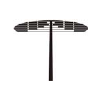## Articles inPress

1. A Generalized Fixed Point Theorem in Fuzzy $b-$Metric Spaces and Applications. M. Dahhouch, N. Makran and B. Marzouki

2. Fractional Order Iterative Boundary Value Problem. M. Menchih, K. Hilal, M. Elomari and A. Kjouni

3. On Some Closed-form Evaluations for the Generalized Hypergeometric Function. A. K. Rathie and B. R. S. Kumar

4. Some Specific Differential Identities in $3$-prime Near-rings. A. Boua and S. Mouhssine

5. A Numerical Calculation of Arc Length and Area Using Some Spline Quasi-interpolants. S. Saidi and A. Abbadi

6. Nontrivial Solutions for a General $p(x)$-Laplacian Robin Problem. F. Kissi and A.R. El Amrouss

7. New Algorithm on Linearization-Discretization Solving Systems of Nonlinear Integro-Differential Fredholm Equations. I. Sedka, S. Khellaf, S. Lemita and M. Z. Aissaoui

8. Orbits of Random Dynamical Systems. A. Zaou, O. Benchiheb and M. Amouch

9. Approximation and Analysis Regarding the Structure of a Multiple Variable Mapping. A. Bodaghi, M. Mosleh and H. Dutta

10. Theta Models for Daily Pandemic Data. M. Acim, M. Zahid and A. Ez-Zetouni

11. Generalizations of 2-absorbing Primal Ideals in Commutative Rings. A. Jaber and R. Shaqbou'a

12. Persistence and Extinction for Stochastic HBV Epidemic Model with Treatment Cure Rate. M. Sadki, A. Ez-zetouni and K. Allali

13. Preservation Theorems of Weakly $\mu\mathcal{H}$-Countably Compact Spaces. A. Qahis and T. Noiri

14. Three Weak Solutions for a Class of Quasilinear Choquard Equations Involving the Fractional $p(x, .)$-Laplacian Operator with Weight. H. Harcha, H. Belaouidel, O. Chakrone and N. Tsouli

15. Three Solutions for a Discrete Fourth-Order Boundary Value Problem with Three Parameters. M. Bohner, G. Caristi, S. Heidarkhani and S. Moradi

16. On Pencil of Bounded Linear Operators on Non-archimedean Banach Spaces. A. El amrani, J. Ettayb and A. Blali

17. Automorphisms of Projective Manifolds. T. Aristide

18. On the Solutions and Behavior of Rational Systems of Difference Equations. E. M. Elsayed and H. S. Gafel

19. Numerical Method of the Exact Control for the Elastic String Problem with Moving Boundary. C. Moraes, M. Rincon and G. Antunes

20. Semi-Delta-Open Sets in Topological Space. K. Singh and A. Gupta

21. On some realizable metabelian $5$-groups. Fouad Elmouhib, Mohamed Talbi and Abdelmalek Azizi

22. Imbeddedness and Direct Sum of Uniserial Modules. A. Hasan, Rafiquddin and M. N. Ali

23. New Parametrization of Bertrand Partner D-curves in $\mathbb{E}^{3}$. S. Tamta and R. Shankar Gupta

24. Existence of Solutions for Boundary Value Problems for First Order Impulsive Difference Equations. L. Bouchal and K. Mebarki and S. G. Georgiev

25. Numerical Modeling of Underground Flow Through Porous Media Using a Finite Volumes Scheme. A. Charhabil, S. Jelti, J. El Ghordaf

26. Nonparametric Estimation of the Copula Function with Bivariate Twice Censored Data. S. Toumi, M. Boukeloua, N. Idiou and F. Benatia

27. Nonlocal Boundary Value Problems for Functional Hybrid Differential Equations Involving Generalized $\omega-$Caputo Fractional Operator. H. Malghi, K. Hilal, Ali El Mfadel and A. Qaffou

28. Multiplicity of Solutions for a Nonlinear Nonlocal Problem with Variable Exponent. A. Bousgheiri and A. Ourraoui

29. Some Common Fixed Point Results on ($\psi, \phi$)-contraction. M. C. Arya, N. Chandra and M. C. Joshi

30. Twisted Hessian Curve Over a Local Ring. A. Chillali, A. Grini and M. B. T. Elhamam

31. Alternative Planes and the Curves on Them. A. Has and B. YÄ±lmaz

32. Comparison Between Classification Algorithms Using a Multiple Correspondence Analysis with Python. L. Ahlam and M. Soundes

33. A Generalization of the Regular Function Modulo $n$. B. Alkhamaiseh

34. Solution of Infinite System of Fourth Order Differential Equations in $\ell_{p}$ Space. T. Jalal and A. H. Jan

35. Calderon's Reproducing Formula for Bessel Wavelet Transforms. C. P. Pandey and Pranami Phukan

36. About a New Nonlinear Integro-Differential Equation of Volterra-Strum-Liouville Type with a Weakly Singular Kernel. D. Mezhoud, M. Benssaad, S. Lemita and A. Khellaf

37. $\mathscr{T}$-Commuting Generalized Derivations on Ideals and Semi-Prime Ideal. Nadeem ur Rehman and Hafedh M. Alnoghashi

38. A Note on a Kirchhoff type Boundary Value Problem Involving Riemann-Liouville Fractional Derivative. M. A. Alyami, H. M. Alhirabi and A. Ghanm

39. Analyticity for the Fractional Navier-Stokes Equations in Critical Fourier-Besov-Morrey Spaces with Variable Exponents. F. Ouidirne, C. Allalou and M. Oukessou

40. A Fixed Point Theorem for Weak ($\psi$ - $\phi$)-Jaggi Type Contraction. Pankaj and Manoj Kumar

41. Stability Analysis of Nonlinear Riemann-Liouville Fractional Differential Equations. S. Yessad, K. Ali Khelil, A. Ardjouni and A. Lachouri

42. Impulsive Fractional Dynamic Equation with Non-local Initial Condition on Time Scales. B. Gogoi, U. K. Saha and B. Hazarika

43. Further Results on the Hop Domination Number of a Graph. D. Anusha, S. Joseph Robin and J. John

44. Global Existence and Blow-up of Solutions for a Class of Steklov Parabolic Problems. A. Lamaizi, A. Zerouali, O. Chakrone and B. Karim

45. Applications of $L_{gfs}$-closed Sets In Mixed Fuzzy Soft Ideal Topological Spaces. M. M. Hoque, J. Chakraborty, B. Bhattacharya and B. C. Tripathy

46. Common Fixed Point and Common Coupled Fixed Point Theorems for Weakly Monotone Mappings. E. Prajisha and P. Shaini

47. Three weak solutions for a class of fourth order $p(x)$-Kirchhoff type problem with Leray-Lions operators. K. Soualhine, M. Filali, M. Talbi and N. Tsouli

48. Derivation Alternator Rings with $S(a,b,c) = 0$. Dr. P. Sarada Devi, Dr. K. Hari Babu, Dr. Y. Suresh Kumar

49. Stability Analysis of a Delayed SEIRQ Epidemic Model with Diffusion. E. Anaama, C. Allalou and K. Hilal